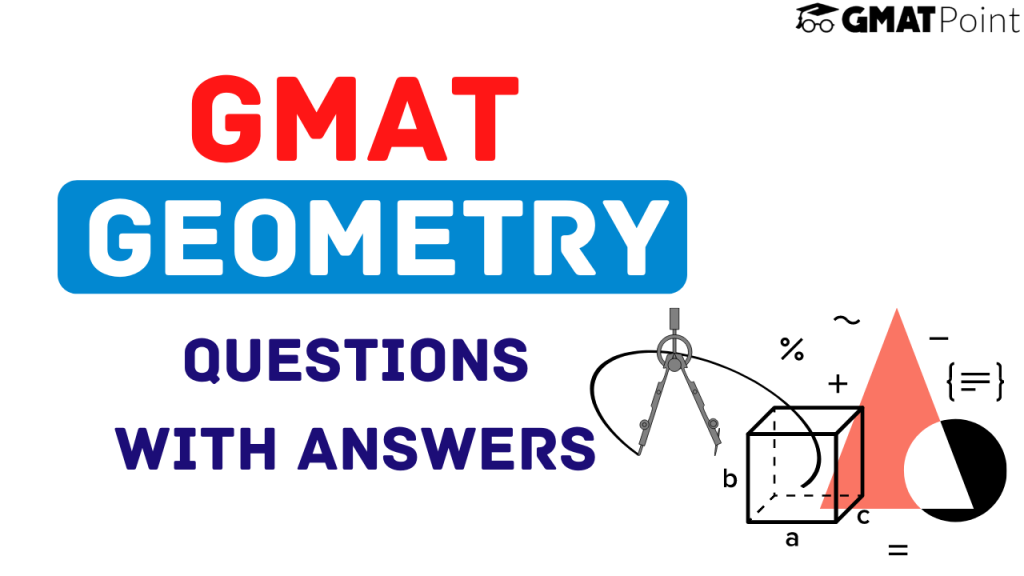# GMAT Geometry Questions With Answers# GMAT Geometry Questions With Answers

Geometry questions constitute a major portion of the Quant section of the GMAT. These questions can come under both Problem Solving and Data Sufficiency types.Geometry has a number of topics in it. In this article, we will be looking into –

1. GMAT Geometry Topics
2. GMAT Geometry Questions with Answers

## GMAT Geometry Topics

In the GMAT, Geometry constitutes a number of topics. They are as follows (this list is in accordance with the GMAT Official Guide):

1. Lines and Angles
2. Convex Polygons
3. Triangles
5. Circles
6. Rectangular solids and cylinders
7. Coordinate Geometry

## Examples – GMAT Geometry Questions with Answers and explanations

### Question 1:

If a convex polygon has 5 diagonals, what is the sum of internal angles of the polygon?

### Approach:

The number of diagonals is equal to the total number of lines formed by joining any two vertices minus the number of sides.

Hence, the number of diagonals = nC2 – n = n(n-1)/2 – n = n(n-3)/2 = 5

n(n-3) = 10

n = 5

Hence, the sum of internal angles = (5-2) x 180 = 540 degrees.

### Question 2:

The length of the equal sides of an isosceles triangle is 6 cm. What is the maximum possible area of the triangle(in square centimetres)?

### Approach:

Let us assume that one of these 6 cm sides is the base and the angle that the other 6 cm side makes with the base is k degrees.

Now, we know that the area of a triangle whose 2 adjacent sides are known and the enclosed angle is known can be calculated as:

Area = (1/2)*a*b*sin(k)

a = First side

b = Second side

k is the angle enclosed.

Now, a and b are fixed as 6 cm each

If sin k is maximum = 1, the value will be maximum.

Hence, the maximum possible area = (1/2) x 6 x 6 = 18

### Question 3:

What is the radius of a circle C?

Statement 1: The ratio of the circumference and the diameter = 3.14

Statement 2: The ratio of the area and the circumference is 5.4 units.

Approach:

Statement 1: Circumference = 2 * pi * r

Diameter = 2 * r

Ratio = pi

The statement does not add any value to the information that is provided.

Statement 2: The ratio of the area and the circumference is 5.4 units.

pi * r * r / (2 * pi * r) = 5.4

r = 10.8 units.

Hence, Option B – Statement 1 alone is not sufficient, Statement 2 alone is sufficient is the correct choice.

### Note:

Though these examples provide a good sense of what type of GMAT Geometry questions you can expect, in no way do they represent the exhaustive list of concepts required for the Quantitative section of GMAT.

### Tips to keep in mind:

1. Try to take some time out from the Problem-Solving Geometry questions so that you can use that time to solve the tricky Data Sufficiency Geometry questions.
2. Do not get stuck in a question for long. If you find yourself trapped in a question for long, take a guess and move on.
3. Read the units(if any) carefully.
4. Look out for negation words. For example: Which of the following are NOT possible values of x?
5. Some questions can be solved faster by the use of options. Make sure you don’t solve these questions in a conventional way.

You can check out the Free GMAT Daily Targets on our platform.

Also, check out the Free GMAT Verbal Tests and Quant Tests.

If you are starting your GMAT preparation from scratch, do check out GMATPOINT.